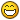## Recommended Posts

I have this code. Hi purpose is to give me random position of spikes. Each spike is 50 px height.

for(var i = 0; i < 6; i++){

var group = this.rightSideSpikes.create(Bird.GAME_WIDTH-30, this.spikesPositions(), "rightSideSpikes");

group.body.immovable = true;

group.body.setSize(22, 17, 8, 17);

}

},

spikesPositions: function(){

var pos = [100, 160, 220, 280, 340, 400];

return pos[Math.round(Math.random() * pos.length-1)];

},

Sometimes it generates spike at the position GAME_WIDTH,0 and I don't know why.

##### Share on other sites

I have this code. Hi purpose is to give me random position of spikes. Each spike is 50 px height.

for(var i = 0; i < 6; i++){
var group = this.rightSideSpikes.create(Bird.GAME_WIDTH-30, this.spikesPositions(), "rightSideSpikes");
group.body.immovable = true;
group.body.setSize(22, 17, 8, 17);
}
},

spikesPositions: function(){
var pos = [100, 160, 220, 280, 340, 400];
return pos[Math.round(Math.random() * pos.length-1)];
},

Sometimes it generates spike at the position GAME_WIDTH,0 and I don't know why.

`Math.round(Math.random() * pos.length-1)`

can return -1 and pos[-1] is undefined.

`// Returns a random number between min (inclusive) and max (exclusive)function getRandomArbitrary(min, max) {  return Math.random() * (max - min) + min;}`

https://developer.mozilla.org/en-US/docs/Web/JavaScript/Reference/Global_Objects/Math/random

##### Share on other sites

Actually, I don't get it...

randomPos: function(min, max){
return Math.random() * (max - min) + min;
},

spikesPositions: function(){
var pos = [100, 150, 200, 250, 300, 350, 400];
return pos[this.randomPos(1, 6)];
}

I've always had problems with this kind of stuff. Even at college##### Share on other sites

Actually, I don't get it...

randomPos: function(min, max){
return Math.random() * (max - min) + min;
},

spikesPositions: function(){
var pos = [100, 150, 200, 250, 300, 350, 400];
return pos[this.randomPos(1, 6)];
}

I've always had problems with this kind of stuff. Even at collegeLook

`randomPos: function(min, max){        return Math.random() * (max - min) + min;    },        spikesPositions: function(){        var pos = [100, 150, 200, 250, 300, 350, 400];        return pos[Math.round(this.randomPos(1, 6))];    }`

Because

`Math.random() * (max - min) + min;`

returns float.

##### Share on other sites

Phaser does have some very handy utilities to make this easier; you could write the above as:

`    spikesPositions: function() {        return this.game.rnd.pick([100, 160, 220, 280, 340, 400]);        // this could also be written as Phaser.Math.getRandom([100, 160, 220, 280, 340, 400]);        // or the mathematical way: 100 + (Math.floor(Math.random() * 6) * 60);    },`

Take a look at the Phaser.Math, Phaser.RandomDataGenerator and Phaser.Utils objects for some nice time-saving stuff.

##### Share on other sites

I think it is impossible that Phaser has pretty much everything what I need to create a simple game.So easy solution. Thank you again, lewster.